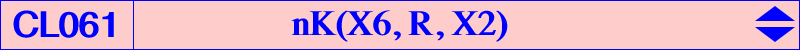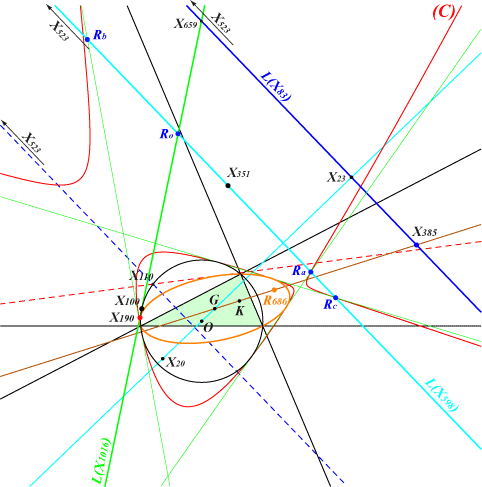CL061 is the class of isogonal nKs passing through the centroid G and the Lemoine point K. They form a net of cubics that contains many special cubics. If the root R is u:v:w, the equation of the cubic is : ∑ u x (y - z) (c^2 y - b^2 z) = 0 or ∑ a^2 y z [v (x - z) + w (x - y)] = 0. See the related CL062. The table gives a selection of these cubics with root R and centers other than G, K.cubic R centers X(2), X(6) and X(i) for i = remark K017 X(385) 99, 512, 14606, 14607, 14608, 14609 nK0 K018 X(523) 13, 14, 15, 16, 111, 368, 524, 5000, 5001, 11537, 11549, 32618, 32619, 39162, 39163, 39164, 39165, 47072, 47073 nK0, focal K072 X(9969) 3, 4, 542, 842, 6328, 14246, 14355, 14356, 14357, 14366, 38940 focal K107 X(3) 2395, 2421 K231 X(20) 34211, 34212 K249 X(14610) 17, 18, 61, 62, 14658, 32525 focal K250 X(9131) 371, 372, 485, 486, 6792, 14645, 14659 focal K383 X(14543) 1, 3, 4, 3945, 41501, 45926 cK K384 X(14544) 3, 4, 9, 40, 57, 84, 14550, 14551, 14552, 14553 K385 X(14546) 3, 4, 7, 55, 672, 673, 942, 943 K386 X(14545) 3, 4, 8, 56, 104, 517, 1193, 1220, 45998 K397 X(30) 110, 523, 5967, 5968, 14998, 14999 K505 X(14511) 13, 14, 15, 16, 30, 74, 378, 3580, 4846, 14910 K506 X(14512) 7, 8, 21, 55, 56, 65, 672, 673, 1193, 1220, 4185, 34259 K507 X(14513) 31, 32, 39, 75, 76, 83, 2276, 14619, 14620, 14621, 14622, 14623, 17750 K686 X(14614) 34203, 34204 equilateral K688 X(351) 5108, 5969, 5970, 14948, 39641, 39642, Brocard points focal K689 X(1633) 1, 241, 294, 948, 949, 2303, 6203, 6204, 7347, 7348, 41356 cKClassification by type When the root lies on a certain locus (L), the cubic (K) = nK(X6, R, X2) has a specific characterization as shown in the next table and the figure below. L(M) denotes the trilinear polar of M.(L) characterization examples circumcircle (K) decomposes into a line through K and its isogonal transform Steiner ellipse (K) decomposes into a line through G and its isogonal transform L(X83) = 23,385,523... (K) is a nK0 L(X598) = 351,523... (K) is a focal cubic with focus on the circumcircle L(X1016) = 100,190... (K) is a cK with node X(1) extraversions of L(X1016) (K) is a cK with node an excenterRemarks : • the line L(X1016) and its extraversions (green lines) are the four common tangents to the circumcircle and the Steiner ellipse. • for any R on the (dashed red) line X107-X110, the cubic (K) contains X(3) and X(4) and the tangents at these points concur at X(6). • for any R on the (dashed blue) line X110-X523, the cubic (K) contains X(13), X(14), X(15), X(16). Further details below. • when R is one of the intersections Ro, Ra, Rb, Rc of L(X598) with L(X1016) and extraversions, the cubic is a strophoid with node X(1) and excenters respectively. *** For any R on the (red) cubic (C), (K) has three concurring asymptotes. No ETC center was found on (C).Classification by centers Any cubic (K) = nK(X6, R, X2) that contains a center P (which is not G, K or an in/excenter) must contain the isogonal conjugate P* of P, P1 = GP /\ KP*, P2 = KP /\ GP* = P1*. It follows that the cubics passing through P have 9 common points hence they belong to a same pencil whose root R lies on a certain line (L). (L) contains the trilinear poles of the lines GP, GP* (on the Steiner ellipse) and KP, KP* (on the circumcircle) giving in general four decomposed cubics of the pencil. When R traverses (L), the tangents at P, P* to (K) intersect on the circum-conic passing through P and P*. Note that the four points P, P*, P1, P2 are not necessarily all distinct : • P = P1 (therefore P* = P2) if and only if P lies on the Grebe cubic K102, in which case the tangents at P, P* concur at G. • P = P2 (therefore P* = P1) if and only if P lies on the Thomson cubic K002, in which case the tangents at P, P* concur at K. In both cases, (K) has fixed tangents at P and P* for any root R on (L). This occurs in the yellow lines of the table.P P*, P1, P2 centers on (L) examples X(3) X(4) X107, X110, X648 X(7) X(55), X(672), X(673) X101, X514, X664, X666, X927, X3234 X(8) X(56), X(1193), X(1220) X109, X190 X(9) X(57) X100, X658, X664, X934, X2283, X2398 K384 X(13) X(14), X(15), X(16) X110, X476, X523, X3233 X(17) X(18), X(61), X(62) X110, X930 K249 X(30) X(74), X(378), X(4846) X648, X1302, X1304 K505 X(31) X(75), X(2276), X(2276)* X101, X668, X789 K507 X(32) X(39), X(76), X(83) X110, X670, X689 K507 X(110) X(523), X(5967), X(5968) X30, X98, X671, X691, X2080, X2966 K397 X(371) X(372), X(485), X(486) X110, X925 K250Note : the cubics corresponding to the first line of the table are also members of the class CL062. *** The transformations f1 : P -> P1 = GP /\ KP* and f2 : P -> P2 = KP /\ GP* = P1* are closely related to the Cundy-Parry transformations as in CL037. Indeed, these are also involutions with singular points A, B, C, G, K. f1 leaves any nK(X6, R, X2) globally invariant and fixes the Thomson cubic K002 and the Grebe cubic K102. Moreover, any cubic of the pencil generated by K002, K102 is transformed into another cubic of the same pencil. These two cubics are isogonal pKs and their pivots are harmonically conjugated with respect to G and K. f1 transforms a line (L) not passing through G and K into a nodal circum-cubic (K) with node G passing through K and f2 transforms the same line (L) into the isogonal transform of the cubic. For example, when (L) is the line at infinity, these two cubics are K280 and K281. If S is the trilinear pole of (L), then • (K) is a K0 when S lies on the circum-hyperbola through G and K in which case (L) contains X(512) i.e. (L) is perpendicular to the Brocard axis. • (K) is a nK when S lies on the circumcircle or on the Steiner ellipse (these two giving decomposed nKs) or on the circum-conic with perspector X(39). In this case, (K) is an isotomic nK with root on the line passing through X99, X670, X804, X874, X880, X887, X1632, X1634. In particular, with S = X(694), the cubic is cK0(#X2, X804), the only cubic of this type. • (K) is a cuspidal cubic when S lies on the isogonal transform of the Kiepert parabola in which case (L) is tangent to the circum-hyperbola through G and K. • the nodal tangents are perpendicular when the X(1992-isoconjugate of S lies on the line X(2), X(39). • there is only one point S for which (K) is circular (resp. equilateral). In both cases, S is complicated and (K) is not particularly interesting.